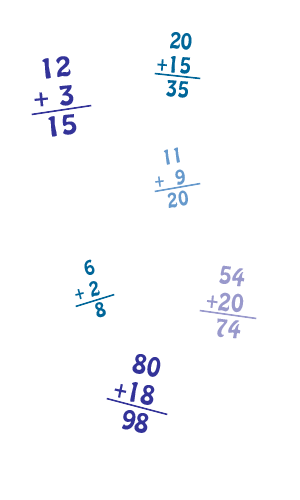6 + 9 = 3 + 1 = 8 + 7 = 3 + 5 = 10 + 9 = 3 + 10 =
Numbers from 1 to 10 1 to 20 1 to 50 1 to 100
 Additions | Subtractions | Illustrated words | Puzzle of letters | Writing quiz | Audio quiz | Tic-Tac | What's the time
 | | | | | © 2010-2012 legangduyeti.com